# How are Fibonacci numbers expressed in nature

### Fibonacci numbers

Fibonacci sequences: These are consequences (an)n real numbers, which are defined as follows:

Be a0, a1 real numbers.
Define inductively for n ≥ 2

an + 1 = an + an-1 for n ≥ 1

Expressed differently: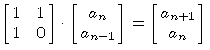Now be a0 = 0, a1 = 1.

Question: For example, how big is a40? or also a300 ?

It is a40 = 102.334.155 = 3 x 5 x 7 x 11 x 41 x 2161
And a300= 222232244629420445529739893461909967206666939096499764990979600

For n ≥ 1 let

 v (n) =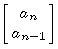So this is a vector in R.2.
Here are the first 8 vectors v (1), ..., v (8):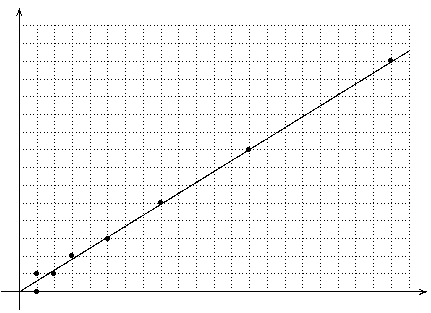They are all close to the straight line with an incline(You can formulate this as follows: the given value is just lim an-1/ an)
and indeed they "commute" around this straight line - why?

We operate here with the matrix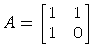on the layer R.2. The following applies:The following calculation rules apply to the eigenvalues: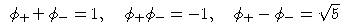It is advisable to also consider the following vector: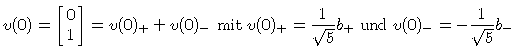So it holds for all n ≥ 0: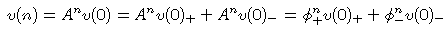because

• v (0)+ is an eigenvector with eigenvalue φ+,
• v (0)- is an eigenvector with eigenvalue φ-,

If we only consider the first coordinate (the x-coordinate) of v (n), we get: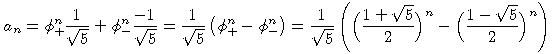This is called the Binet's formula (he published it in 1842, but it was already known to Euler and D. Bernoulli).

Here, for clarification, the vectors v (0), ..., v (4):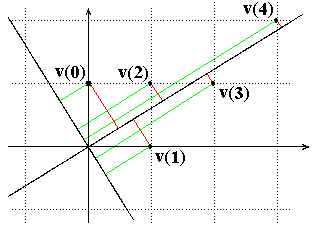The two inclined straight lines are the natural lines of the matrix A.
For each of the vectors v (0), ..., v (4) it is shown how it is the sum of a vector in the eigenline g+ = R.b+ and a vector in the eigenline g- = R.b- writes:
v (n) = v (n)++ v (n)- with v (n)+ in g+ and v (n)- in g-
(The green line is (parallel to) v (n)+, the red is (parallel to) v (n)-.) And the following applies:
v (n + 1)+ = λ+v (n)+ and v (n + 1)- = λ-v (n)-
The length of the vectors v (n)- converges to 0, so v (n) differs from v (n)+ for large n only very little.

Since one knows that v (n) is an integer vector, one can in this way v (n) exactly to calculate! We are only interested in the x-coordinate of v (n), because this is the i-th Fibonacci number an.